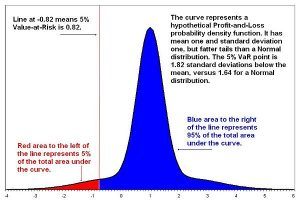21
Apr 18

## Value at Risk and its calculation for a normal distribution

Variance is a symmetric measure of risk. Positive and negative values of the measured variable equally influence variance. Value at Risk is an asymmetric measure of risk and is more useful when negative values, like losses of a bank, are of concern. The bank management can ask a question: What is the probability of us losing today 99% of our assets or more? If$L$ is the loss, as percentage of total assets, and it takes negative values, as in accounting books, this question is written as: How much is$P(L\le -0.99)$? Here the bound for losses is fixed and the probability is sought.

## Value at Risk definition

The Value at Risk approach asks an opposite question. It recognizes that bad things happen and attempts to control the size of a loss fixing the probability of a loss. That is, the question is: How much is at risk, given the probability$\alpha$% of loss? This translates to

(1)$P(L\le VaR)=\alpha$.

Value at Risk (VaR) is the solution to this equation (it is defined implicitly by this equation). The left side of the equation is recognized as the value of the distribution function of$L$ at point VaR, so the equation becomes

(2)$F_L(VaR)=\alpha$.

When$F_L$ has an inverse, this equation is equivalent to

(3)$VaR=F_L^{-1}(\alpha)$.

Thus, in this case finding VaR is as simple as inverting a function. Once again, (1), (2) and (3) are different ways to write the same thing. See illustration in Figure 1.

Remark. If$L$ takes negative values with positive probability, then for small$\alpha$ VaR is negative. VaR sometimes is reported as a positive number. In that case people use the definition$P(L\le -VaR)=\alpha$ or, alternatively,$VaR=-F_L^{-1}(\alpha)$.Figure 1. VaR diagram

## Case of a normally distributed return

Suppose return$R$ on a portfolio is normally distributed,$R\sim N(\mu,\sigma^2)$. Then it can be written as

(4)$R=\mu+\sigma z$,

where$z$ is standard normal. The conventional notation for the distribution function of the standard normal is$\Phi$. More precisely,$\Phi(x)=\int_{-\infty}^xp_z(t)dt$, where$p_z(t)=(2\pi)^{-1/2}\exp(-t^2/2)$ is the density of standard normal.

To find the Value at Risk$VaR^\alpha$ corresponding to given$\alpha$ we just apply the definition and equation (4):

(5)$P(R\le VaR^\alpha)=P(\mu+\sigma z\le VaR^\alpha)=P(z\le \frac{VaR^\alpha-\mu}{\sigma})=\Phi\left(\frac{VaR^\alpha-\mu}{\sigma}\right)=\alpha$,

which gives$\frac{VaR^\alpha-\mu}{\sigma}=\Phi^{-1}(\alpha)$

or

(6)$VaR^\alpha=\mu+\sigma\Phi^{-1}(\alpha)$.

In a time series context, if we have a series of returns, similarly to (4) we can assume that at each point in time$R_{t+1}=\mu_{t+1}+\sigma_{t+1} z_{t+1}$,

where$z_{t+1}$ is standard normal conditional on the information set$\mathcal{F}_t$:$z_{t+1}\vert\mathcal{F}_t\sim N(0,1)$.

The calculation is essentially the same as in (5), just put subscripts everywhere and make the probability conditional on$\mathcal{F}_t$ (that gives the conditional VaR). The solution is obtained from (6) by putting subscripts:

(7)$VaR^\alpha_{t+1}=\mu_{t+1}+\sigma_{t+1}\Phi^{-1}(\alpha)$.